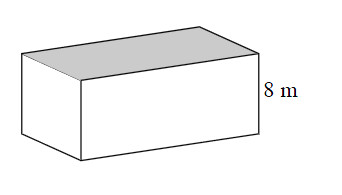### Home > MC1 > Chapter 9 > Lesson 9.3.2 > Problem9-141

9-141.

In the right prism shown at right, the area of the base (shaded) is $42.5$ square meters. Find the volume of the prism.

The volume of the prism is equal to the area of the base times the height.

Erik is trying to find the volume of a similar prism. The base has an area of $50$ square feet and a height of $10$ feet.
He finds that the volume is $500$ cubic feet, since $\left(50 \text{ sq ft}\right)\left(10 \text{ ft}\right) = 500$ cubic ft.